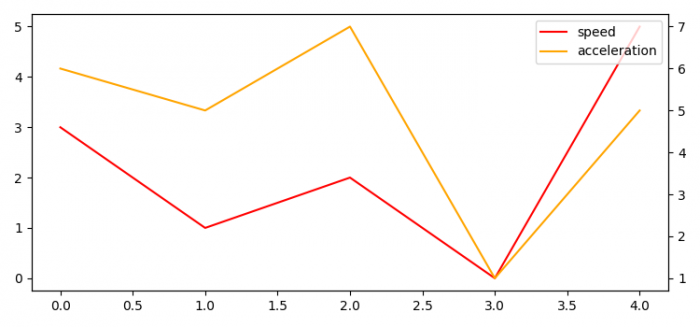# How to plot single data with two Y-axes (two units) in Matplotlib?

To plot single data with two Y-Axes (Two units) in Matplotlib, we can take the following steps −

• Set the figure size and adjust the padding between and around the subplots.
• Create speed and acceleration data points using numpy.
• Add a subplot to the current figure.
• Plot speed data points using plot() method.
• Create a twin Axes sharing the X-axis.
• Plot acceleration data point using plot() method.
• Place a legend on the figure.
• To display the figure, use show() method.

## Example

import matplotlib.pyplot as plt
import numpy as np

plt.rcParams["figure.figsize"] = [7.50, 3.50]
plt.rcParams["figure.autolayout"] = True

speed = np.array([3, 1, 2, 0, 5])
acceleration = np.array([6, 5, 7, 1, 5])

ax1 = plt.subplot()
l1, = ax1.plot(speed, color='red')
ax2 = ax1.twinx()
l2, = ax2.plot(acceleration, color='orange')

plt.legend([l1, l2], ["speed", "acceleration"])

plt.show()

## Output